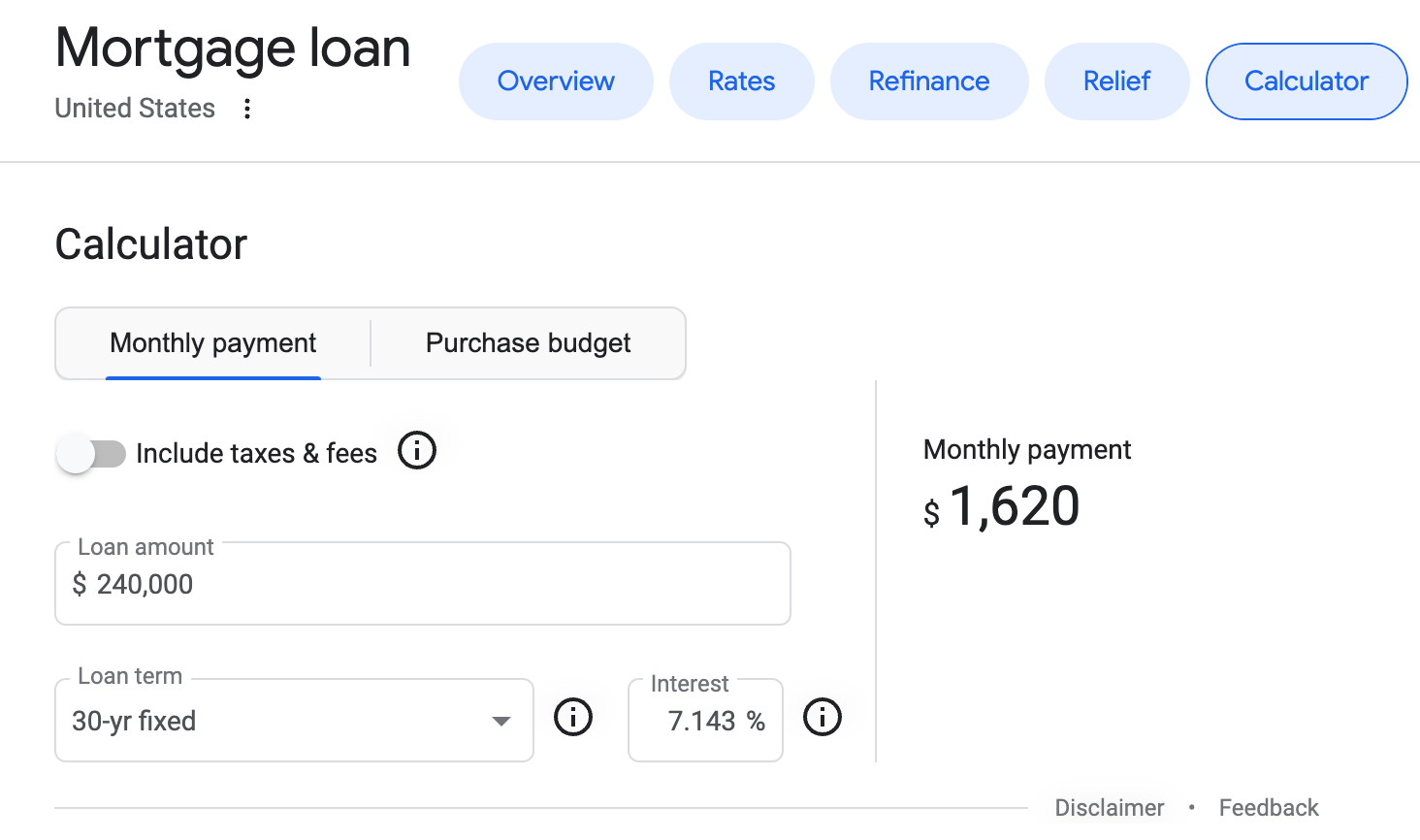# Mortgage Calculator

AuthorLanguages
HTMLJS
Difficulty
Easy
Recommended Duration
20 mins
Users Completed

Build a simple mortgage calculator widget that takes in a loan amount, interest rate, loan term, and calculates the monthly mortgage payment, total payment amount, and total interest paid.

## Requirements

• The user should be able to enter:
• Loan amount (\$)
• Annual interest rate (%). This is also known as the annual percentage rate (APR)
• Loan term (in years)
• Using the inputs, the calculator should compute the following and display the results to the user:
• Monthly mortgage payment
• Total payment amount
• Total interest paid
• If a non-numerical string is entered into any input field, the calculator should display an error message. Additionally, the calculator should handle any other invalid inputs that may arise.
• Round the result amounts to 2 decimal places.

The last two requirements might not be given to you during interviews, you're expected to clarify.

The formula for calculating the monthly payment is:

M = P(i(1+i)n)/((1+i)n - 1)

• M: Monthly mortgage payment
• P: Loan amount
• i: Monthly interest rate (APR / 12)
• n: Total number of payments (loan term in years x 12)

Here's an example of Google's mortgage calculator (you might need to be in the US for it to appear):## Source

This question is adapted from FrontendEval's Mortgage Calculator.